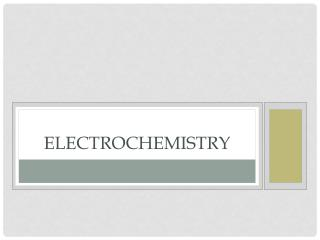DownloadDownload PresentationElectrochemistry

# Electrochemistry

Télécharger la présentation## Electrochemistry

- - - - - - - - - - - - - - - - - - - - - - - - - - - E N D - - - - - - - - - - - - - - - - - - - - - - - - - - -
##### Presentation Transcript

1. Electrochemistry

2. Redox reactions • Write the balanced equation for the reaction of permanganate and oxalate in acidic solution. • MnO4- + C2O42- Mn2+ + CO2 • 1. Find oxidation numbers

3. Permanganate & oxalate • Separate into half reactions • Balance for mass, balance O with H2O, balance H with H+, balance charge w/ electrons

4. Finishing the problem • Balancing the electrons • Combine and cancel

5. Redox titration • Use the ratio from the balanced redox reaction to determine concentrations. • How will we know when the reaction is complete? • We use an indicator • In our earlier redox reaction, permanganate is a “self indicator”.

6. Activity series • Some substances are more easily oxidized than others. • Listing these in order of their ability to oxidize gives us an activity series. • A substance will oxidize if its above the item reducing it on the table. • Cu will be oxidized by Ag+ because its higher on the activity series. Ag cannot be oxidized by Cu2+.

7. Galvanic Cell • Using a spontaneous redox reaction to generate electricity. • Anode = oxidation (AnOx) • Reduction at the cathode (RedCat) • Electrons flow From Anode To Cathode (FatCat)

8. Salt Bridge • Allows ions to travel between solutions, maintaining charge balance. • Can be a solution, a paste or a porous membrane

9. Galvanic cell notation • A shorthand way of writing the structure of a galvanic cell is • Anode | Anode ion || Cathode Ion | Cathode • | denotes a phase barrier (solid metal and aqueous solution) • || denotes the salt bridge • Mg(s) | Mg2+(aq) || Al3+(aq) | Al(s) is the cell notation of an aluminum/magnesium cell

10. Cell potential • The Standard Reduction Potential table measures the voltage produced when that half reaction is reduced by the standard hydrogen electrode • A negative voltage means that electricity must be applied to cause the reaction (more later) • All concentrations are assumed 1M, pressures are 1 atm at 298K. • How does this compare to the activity series?

11. Cell potential of a galvanic cell • Ecell = Ered + Eox • When you flip the oxidation half reaction, change the sign of the voltage!!! • What is the cell potential of a copper/zinc cell?

12. Still more gibbs (and some equilibrium) • ΔG° = -nFE° • n is the number of moles of electrons transferred (from balanced equation) • F is the Faraday constant (~96,500 coulomb/mol) • E° is the standard cell potential

13. Practice • Calculate ΔG° and K from the following reaction: • 4Ag(s) + O2(g) + 4H+(aq)  4Ag+(aq) + 2H2O(l)

14. cell potential at nonstandard conditions • Gibbs at Nonstandard Conditions • ΔG = ΔG° + RT lnQ • Substituting –nFE into the equation gives us the Nernst Equation: At 298K, we can rewrite it as

15. Practical use of nonstandard conditions • Concentration cell • Same anode and cathode • Same electrolyte • E° of the cell is 0. • Due to the huge differences in concentration, Q will not = 1. This gives a small voltage.

16. concentration cell • What voltage will be generated by the cell on the right?

17. Batteries

18. hydrogen Fuel Cells • Hydrogen is oxidized to form H+ and e-. • The electron passes through a wire and performs work. • H+ travels through the membrane, combines with O2 and the electron to form H2O.

19. Corrosion • Most metals allow free flow of electrons. • In the presence of H+, Fe will oxidize to Fe2+. • Water acts as both a source of H+ and the electrolyte for Fe2+ ions to flow. • Further oxidation of Fe2+ and combination with oxygen form Fe2O3.

20. Preventing corrosion • Galvanization • Coating a corrodible metal will prevent corrosion. • Cathodic Protection • Attaching a metal that is easier to oxidize will prevent corrosion. • Ships attach large pieces of magnesium to their hull. The magnesium will oxidize in place of the iron.

21. Electrolysis • Adding electricity to a nonspontaneous reaction will cause it to occur. • We can generate sodium metal and chlorine gas from a molten NaCl sample.

22. predicting electrolytic half reactions • Use your reduction potential table. The combination that produces the most positive voltage will occur. • Beware of aqueous solutions • Water can be oxidized to form O2, or reduced to form H2. You must keep these in mind when figuring out what reactions occur.

23. molten vs aqueous • Molten NaI will form metallic sodium and iodine. Will NaI(aq) do the same? • There are four possible reactions: • Oxidation • 2I- I2 + 2e- E = -0.54V • 2H2O  O2 + 4H+ + 4e- E = -1.23V • Reduction • Na+ + e-  Na E = -2.71V • 2H2O + 2e-  H2 + 2OH- E = -0.83V • Let’s prove it!

24. electroplating • If we have a metallic anode and an electrolyte solution of that metal, we can coat an object (serving as the cathode) with that metal.

25. electroplating stoichiometry • We know that: • 1 mol e- = 96,500 coulombs (Faraday’s Constant) • 1 Amp = 1 coulomb/sec • Electroplating questions are simple factor-label!

26. Example • Calculate the number of grams of aluminum produced in 1.00 hour by the electrolysis of molten AlCl3 at a current of 10.0 A. • Al3+ + 3e- Al